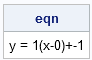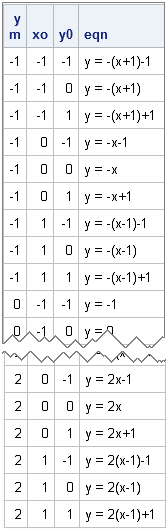I needed to construct a string to use in the title of a scatter plot. The scatter plot showed a line, and I wanted to include the equation of the line in the plot's title. This article shows how to construct a string that contains the equation in a readable form.

There are several ways to represent the equation of a line, but I wanted to use the "point-slope formula," which is useful when you have a line with slope m that passes through the point (x0, y0). The equation of the line can be written in the standard form y = m(x-x0) + y0.

In the SAS/IML language, you can concatenate strings by using the '+' operator, so a simple implementation is as follows:

 proc iml; /* define the parameters, which are numbers */ x0 = 0; y0 = -1; m = 1;   /* convert numbers to strings by using the BEST9. format */ sx0 = strip(putn(x0, "BEST9.")); sy0 = strip(putn(y0, "BEST9.")); sm = strip(putn(m, "BEST9."));   eqn = "y = " + sm + "(x-" + sx0 + ")+" + sy0; print eqn;The sx0, sy0, and m variables are string representations of the numbers. The PUTN function applies the BEST9. format to convert numbers to strings. The STRIP function removes any extraneous blanks from a string.

Although this equation is satisfactory for my own personal use, I wouldn't want to use it in a presentation. An equation that is simpler to read is y = x-1. In particular, I'd like to write a SAS/IML function that creates the equation of a line from the parameters, but handles the following special situations:

• m is –1, 0, or 1
• x0 is 0 or negative
• y0 is 0 or negative

For these special conditions, the equation of a line simplifies or the '+' and '-' symbols need to be modified. Consequently, I wrote the following SAS/IML function, which is a simple exercise in IF-THEN/ELSE logic:

 start GetEqnOfLine(v); /* Build equation of a line in the form y=m(x-x0)+y0 Special cases: m = -1, 0, 1 x0= 0, negative y0= 0, negative */ /* convert numbers to strings by using the BEST9. format */ x0 = v; y0 = v; m = v; sx0 = strip(putn(v, "BEST9.")); sy0 = strip(putn(v, "BEST9.")); sm = strip(putn(v, "BEST9."));   if m=. then return( "x=" + sx0 ); /* m=. means vertical line */   s = "y = "; /* initialize string */   /* Concatenate onto s: add m term unless m=0 */ if sm='-1' then s = s + "-"; else if sm='1' | sm='0' then /* s is unchanged */; else s = s + sm;   /* add (x-x0) term unless m=0. Handle x0=0 and x0<0 */ if sm='0' then /* s is unchanged */; else if sx0='0' then s = s + "x"; else if x0<0 then s = s + "(x+" + substr(sx0,2) + ")"; else s = s + "(x-" + sx0 + ")";   /* add +y0 term. Handle y0=0 and y0<0 */ if sm='0' then s = s + sy0; else if sy0='0' then /* s is unchanged */; else if y0<0 then s = s + "-" + substr(sy0,2); else s = s + "+" + sy0;   return(s); finish;

The only tricky statements are the ones that call the SUBSTR function. In order to accommodate negative parameters. The SUBSTR function returns the substring of the formatted value beginning at the second position of the string. This omits the leading negative sign.

Incidentally, because all of the quantities in this problem are scalar, you can write a similar function in the SAS DATA step. In the DATA step you can use the '||' operator or the "CAT" series of functions to perform string concatenation.

To test the GetEqnOfLine function, I wrote loops that iterate over various values of the parameters. A helper function calls the SUBPAD function to produce a string of a specified length.

 start BlankString(length); /* return string of a specified length */ return( subpad(" ",1,length) ); finish;   y = j(36,3); /* allocate matrix for parameters */ eqn = j(36,1,BlankString(25)); /* allocate vector for equations */ i = 1; do m = -1 to 2; do x0 = -1 to 1; do y0 = -1 to 1; v = x0 || y0 || m; eqn[i,] = GetEqnOfLine(v); y[i,] = m||x0||y0; i = i+1; end; end; end; print y[c={"m" "xo" "y0"}] eqn;The table is so long that only the beginning and the end are shown.

Although this technique is not computationally challenging, it shows three features that I use repeatedly when I construct strings in SAS:

• Use the '+' operator to concatenate strings in the SAS/IML language.
• Use DATA step functions such as SUBSTR and STRIP to manipulate strings.
• Use the SUBPAD function to generate a character array where each element is a string of a specified length.

After you have constructed the string, it is easy to use the CALL SYMPUT routine to create a macro variable that can be used in a TITLE statement. You can also get fancy with the titles and include Unicode characters to represent Greek letters and superscripts, as shown in an article by Dan Heath.

Share